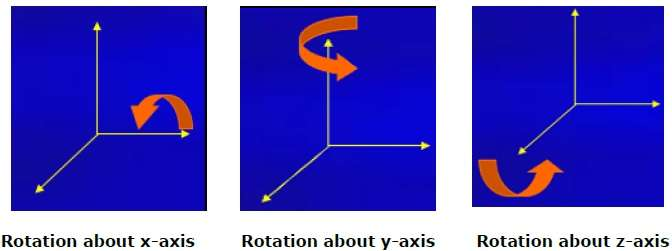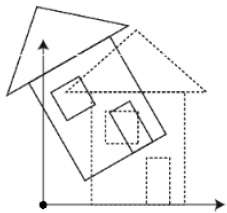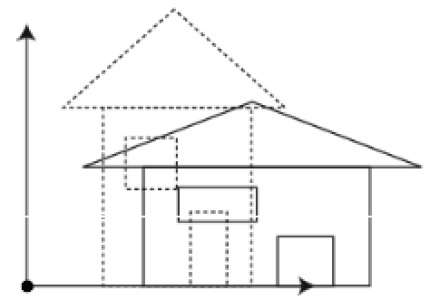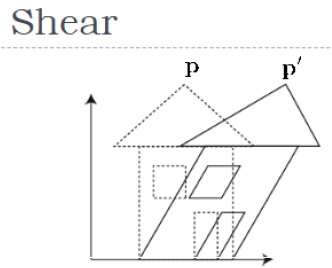# 3D Transformation

## Rotation

3D rotation is not same as 2D rotation. In 3D rotation, we have to specify the angle of rotation along with the axis of rotation. We can perform 3D rotation about X, Y, and Z axes. They are represented in the matrix form as below −

Rx(θ)=⎡⎣⎢⎢⎢10000cosθsinθ00sinθcosθ00001⎤⎦⎥⎥⎥Ry(θ)=⎡⎣⎢⎢⎢cosθ0sinθ00100sinθ0cosθ00001⎤⎦⎥⎥⎥Rz(θ)=⎡⎣⎢⎢⎢cosθsinθ00sinθcosθ0000100001⎤⎦⎥⎥⎥Rx(θ)=[10000cosθ−sinθ00sinθcosθ00001]Ry(θ)=[cosθ0sinθ00100−sinθ0cosθ00001]Rz(θ)=[cosθ−sinθ00sinθcosθ0000100001]

The following figure explains the rotation about various axes −## Scaling

You can change the size of an object using scaling transformation. In the scaling process, you either expand or compress the dimensions of the object. Scaling can be achieved by multiplying the original coordinates of the object with the scaling factor to get the desired result. The following figure shows the effect of 3D scaling −In 3D scaling operation, three coordinates are used. Let us assume that the original coordinates are (X, Y, Z), scaling factors are (SX,SY,Sz)(SX,SY,Sz) respectively, and the produced coordinates are (X’, Y’, Z’). This can be mathematically represented as shown below −

S=⎡⎣⎢⎢⎢⎢Sx0000Sy0000Sz00001⎤⎦⎥⎥⎥⎥S=[Sx0000Sy0000Sz00001]

P’ = P∙S

[XYZ1]=[XYZ1]⎡⎣⎢⎢⎢⎢Sx0000Sy0000Sz00001⎤⎦⎥⎥⎥⎥[X′Y′Z′1]=[XYZ1][Sx0000Sy0000Sz00001]

=[X.SxY.SyZ.Sz1]=[X.SxY.SyZ.Sz1]

## Shear

A transformation that slants the shape of an object is called the shear transformation. Like in 2D shear, we can shear an object along the X-axis, Y-axis, or Z-axis in 3D.As shown in the above figure, there is a coordinate P. You can shear it to get a new coordinate P’, which can be represented in 3D matrix form as below −

Sh=⎡⎣⎢⎢⎢⎢1shxyshxz0shyx1shyz0shzxshzy100001⎤⎦⎥⎥⎥⎥Sh=[1shxyshxz0shyx1shyz0shzxshzy100001]

P’ = P ∙ Sh

X=X+ShyxY+ShzxZX′=X+ShxyY+ShxzZ

Y=ShxyX+Y+shzyZY′=ShyxX+Y+shyzZ

Z=ShxzX+ShyzY+ZZ′=ShzxX+ShzyY+Z

## Transformation Matrices

Transformation matrix is a basic tool for transformation. A matrix with n x m dimensions is multiplied with the coordinate of objects. Usually 3 x 3 or 4 x 4 matrices are used for transformation. For example, consider the following matrix for various operation.

 T=⎡⎣⎢⎢⎢⎢100tx010ty001tz0001⎤⎦⎥⎥⎥⎥T=[100001000010txtytz1] S=⎡⎣⎢⎢⎢⎢Sx0000Sy0000Sz00001⎤⎦⎥⎥⎥⎥S=[Sx0000Sy0000Sz00001] Sh=⎡⎣⎢⎢⎢⎢1shxyshxz0shyx1shyz0shzxshzy100001⎤⎦⎥⎥⎥⎥Sh=[1shxyshxz0shyx1shyz0shzxshzy100001] Translation Matrix Scaling Matrix Shear Matrix Rx(θ)=⎡⎣⎢⎢⎢10000cosθsinθ00−sinθcosθ00001⎤⎦⎥⎥⎥Rx(θ)=[10000cosθ−sinθ00sinθcosθ00001] Ry(θ)=⎡⎣⎢⎢⎢cosθ0−sinθ00100sinθ0cosθ00001⎤⎦⎥⎥⎥Ry(θ)=[cosθ0sinθ00100−sinθ0cosθ00001] Rz(θ)=⎡⎣⎢⎢⎢cosθsinθ00−sinθcosθ0000100001⎤⎦⎥⎥⎥Rz(θ)=[cosθ−sinθ00sinθcosθ0000100001] Rotation Matrix